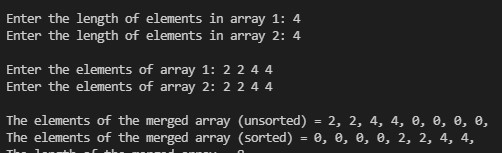# How to join two Vectors using STL in C++?

Given two vectors, join these two vectors using STL in C++.
Example:

Input:
vec1 = {1, 45, 54, 71, 76, 12},
vec2 = {1, 7, 5, 4, 6, 12}
Output: {1, 4, 5, 6, 7, 12, 45, 54, 71, 76}
Input:
vec1 = {1, 7, 5, 4, 6, 12},
vec2 = {10, 12, 11}
Output: {1, 4, 5, 6, 7, 10, 11, 12}

Approach: Joining can be done with the help of set_union() function provided in STL.
Syntax:

```set_union (InputIterator1 first1, InputIterator1 last1,
InputIterator2 first2, InputIterator2 last2,
OutputIterator result);```

## CPP

 `// C++ program to join two Vectors` `// using set_union() in STL`   `#include ` `using` `namespace` `std;`   `int` `main()` `{` `    ``// Get the vector` `    ``vector<``int``> vector1 = { 1, 45, 54, 71, 76, 12 };` `    ``vector<``int``> vector2 = { 1, 7, 5, 4, 6, 12 };`   `    ``// Sort the vector` `    ``sort(vector1.begin(), vector1.end());` `    ``sort(vector2.begin(), vector2.end());`   `    ``// Print the vector` `    ``cout << ``"First Vector: "``;` `    ``for` `(``int` `i = 0; i < vector1.size(); i++)` `        ``cout << vector1[i] << ``" "``;` `    ``cout << endl;`   `    ``cout << ``"Second Vector: "``;` `    ``for` `(``int` `i = 0; i < vector2.size(); i++)` `        ``cout << vector2[i] << ``" "``;` `    ``cout << endl;`   `    ``// Initialise a vector` `    ``// to store the common values` `    ``// and an iterator` `    ``// to traverse this vector` `    ``vector<``int``> v(vector1.size() + vector2.size());` `    ``vector<``int``>::iterator it, st;`   `    ``it = set_union(vector1.begin(),` `                   ``vector1.end(),` `                   ``vector2.begin(),` `                   ``vector2.end(),` `                   ``v.begin());`   `    ``cout << ``"\nAfter joining:\n"``;` `    ``for` `(st = v.begin(); st != it; ++st)` `        ``cout << *st << ``", "``;` `    ``cout << ``'\n'``;`   `    ``return` `0;` `}`

Output:

```First Vector: 1 12 45 54 71 76
Second Vector: 1 4 5 6 7 12

After joining:
1, 4, 5, 6, 7, 12, 45, 54, 71, 76,```

Note that the vector should not contain 1 element twice:In case you enter same elements, the set_union function will only consider 1 instance of the element, and default rest to 0.

Whether you're preparing for your first job interview or aiming to upskill in this ever-evolving tech landscape, GeeksforGeeks Courses are your key to success. We provide top-quality content at affordable prices, all geared towards accelerating your growth in a time-bound manner. Join the millions we've already empowered, and we're here to do the same for you. Don't miss out - check it out now!

Previous
Next